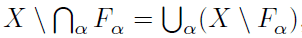# Pure math symbols defined

• B
looking at notes on functional analysis for self study. the authors I have stumbled across so far launch straight into the formal theory. I can't find any middle ground of intuitive motivations for the theory as yet. I am assuming the following is part of set theory? I can't follow the arguments because I don't know the terminology. are there lists defined for example the symbols in the following. I do not want these particular ones defined I want to know how to do it myself.

what branch of math do I need to read to get a foundation in this language.Mark44
Mentor
The \ symbol is "set difference" so A \ B = A ∩ BC (the elements of A intersected with the complement of B)

α Fα means ##F_{\alpha_1} \cap F_{\alpha_2} \cap \dots F_{\alpha_n} \cap \dots##, where the ##\alpha_i##s are some indexing set.
α Fα is similar, but is the union over some indexing set.

Books on analysis usually define these symbols in an appendix.

micromass
Staff Emeritus
Homework Helper
α Fα means ##F_{\alpha_1} \cap F_{\alpha_2} \cap \dots F_{\alpha_n} \cap \dots##, where the ##\alpha_i##s are some indexing set.

Correct, but this gives the impression that the number of ##F_\alpha## is countable, while it can be much more general. In any case, it seems the OP will need to look at some basic set theory before he can handle analysis.

Mark44
Mentor
Correct, but this gives the impression that the number of ##F_\alpha## is countable, while it can be much more general.
Yes, I realize that the ##\alpha##'s are some indexing set, not necessarily a countable set. My intent was to expand the notation somewhat.

Does analysis solve problems or just prove theorems and provide tools for solving problems?

ETA, I am going to do classical analysis on real numbers first re calculus. Seems like the logical thing and apparently I am already familiar with many of the content eg limits, series, real numbers etc.

Then set theory/logic then analysis.

Sound sound?